# findobj

Find graphics objects with specific properties

## Syntax

``h = findobj``
``h = findobj(prop,value)``
``h = findobj('-not',prop,value)``
``h = findobj(prop1,value1,oper,prop2,value2)``
``h = findobj('-regexp',prop,expr)``
``h = findobj('-property',prop)``
``h = findobj(prop1,value1,...,propN,valueN)``
``h = findobj(objhandles,___) ``
``h = findobj(objhandles,'-depth',d,___)``
``h = findobj(objhandles,'flat',___) ``

## Description

example

````h = findobj` returns the graphics root object and all of its descendants.```

example

````h = findobj(prop,value)` returns all objects in the hierarchy that have their property `prop` set to `value`.```

example

````h = findobj('-not',prop,value)` returns all objects whose specified property is not set to the specified value.```

example

````h = findobj(prop1,value1,oper,prop2,value2)` applies the logical operator `oper` to the `prop,value` pairs. For example, `h = findobj('LineStyle','--','-and','Marker','o')` returns all objects that have a dashed line style and circular markers.```

example

````h = findobj('-regexp',prop,expr)` uses a regular expression to find objects with specific property values. Objects with property values satisfying the regular expression are returned.```

example

````h = findobj('-property',prop)` returns all objects that have the specified property.```

example

````h = findobj(prop1,value1,...,propN,valueN)` returns all objects in the hierarchy that have the specified properties set to the specified values. You can replace `prop,value` pairs with other input argument combinations from the previous syntaxes. For example, ```h = findobj(prop1,value1,'-not',prop2,value2,'-property',prop3)``` returns all objects that satisfy these three conditions: The object has a property `prop1` set to `value1`.The object has a property `prop2` whose value is not set to `value2`.The object has a property `prop3`. ```

example

````h = findobj(objhandles,___) ` restricts the search to the objects listed in `objhandles` and all of their descendants. You can restrict the search for any of the previous syntaxes.```

example

````h = findobj(objhandles,'-depth',d,___)` restricts the search to the objects listed in `objhandles` and their descendants that are up to `d` levels lower in the graphics object hierarchy.```

example

````h = findobj(objhandles,'flat',___) ` restricts the search to the objects listed only in `objhandles`. The descendant objects are not searched. Using the `'flat'` option is the same as using the `'-depth'` option with `d = 0`.```

## Examples

collapse all

Delete all existing figures, and then create a plot of random values.

```close all plot(rand(5))```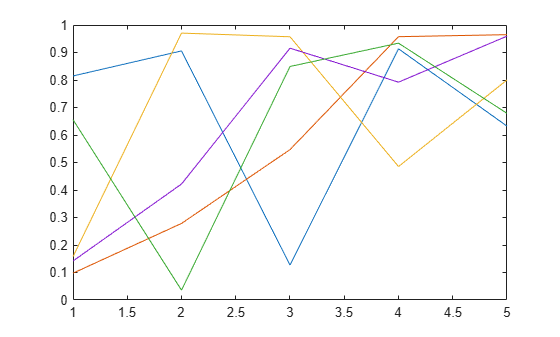Return the graphics root object and all of its descendants.

`h = findobj`
```h = 8x1 graphics array: Root Figure (1) Axes Line Line Line Line Line ```

Delete all existing figures, and then create a multiline plot.

```close all plot(magic(4))```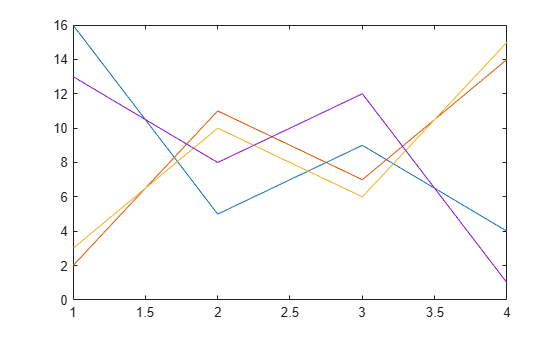Return all line objects.

`h = findobj('Type','line')`
```h = 4x1 Line array: Line Line Line Line ```

Plot nine sine waves with custom colors and line styles.

```x = linspace(0,7); y = ones(length(x),9); for i = 1:9 y(:,i) = sin(x-i/5)'; end plot(x,y) colororder({'red','green','blue'}) ax = gca; ax.LineStyleOrder = {'-','--',':'};```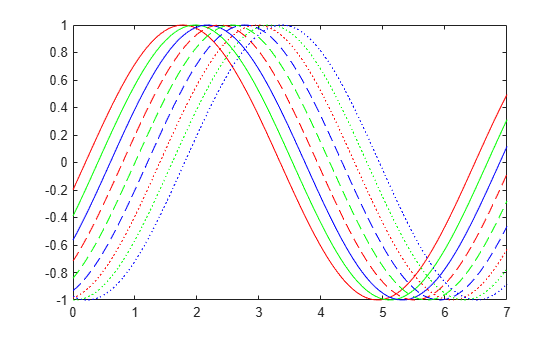Return the solid red line. Then, change the thickness of the line.

`h = findobj('Color','red','LineStyle','-')`
```h = Line with properties: Color: [1 0 0] LineStyle: '-' LineWidth: 0.5000 Marker: 'none' MarkerSize: 6 MarkerFaceColor: 'none' XData: [0 0.0707 0.1414 0.2121 0.2828 0.3535 0.4242 0.4949 0.5657 0.6364 0.7071 0.7778 0.8485 0.9192 0.9899 1.0606 1.1313 1.2020 1.2727 1.3434 1.4141 1.4848 1.5556 1.6263 1.6970 1.7677 1.8384 1.9091 1.9798 2.0505 2.1212 2.1919 2.2626 ... ] YData: [-0.1987 -0.1289 -0.0586 0.0121 0.0827 0.1529 0.2224 0.2907 0.3576 0.4226 0.4856 0.5462 0.6040 0.6588 0.7103 0.7582 0.8024 0.8426 0.8785 0.9101 0.9371 0.9594 0.9769 0.9896 0.9973 1.0000 0.9977 0.9905 0.9782 0.9611 0.9392 0.9126 ... ] Show all properties ```
`h.LineWidth = 2;`Create a multiline plot. Specify an identifier for each plot.

```x = linspace(-1,1); y1 = x; plot(x,y1,'Tag','linear') hold on y2 = x.^2; plot(x,y2,'Tag','quadratic') y3 = exp(x); plot(x,y3,'Tag','exponential') y4 = sin(x); plot(x,y4,'Tag','sinusoidal') hold off```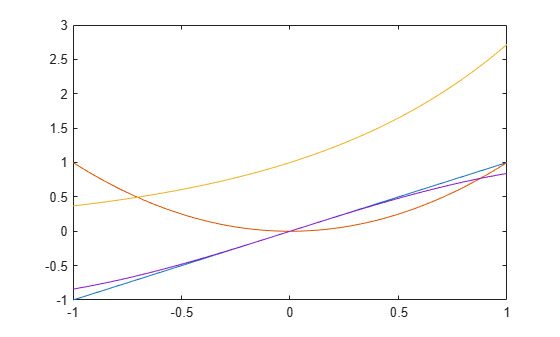Find all objects whose `Tag` property is not set to `'linear'`.

`h1 = findobj('-not','Tag','linear')`
```h1 = 6x1 graphics array: Root Figure (1) Axes Line (sinusoidal) Line (exponential) Line (quadratic) ```

Find all objects whose `Tag` property is not set to `'linear'` or `'quadratic'`.

`h2 = findobj('-not',{'Tag','linear','-or','Tag','quadratic'})`
```h2 = 5x1 graphics array: Root Figure (1) Axes Line (sinusoidal) Line (exponential) ```

Find all line objects whose `Tag` property is not set to `'linear'` or `'quadratic'`.

`h3 = findobj('Type','line','-not',{'Tag','linear','-or','Tag','quadratic'})`
```h3 = 2x1 Line array: Line (sinusoidal) Line (exponential) ```

Improve the readability of the previous statement by using `'-and'` and curly brackets.

`h4 = findobj({'Type','line'},'-and',{'-not',{'Tag','linear','-or','Tag','quadratic'}})`
```h4 = 2x1 Line array: Line (sinusoidal) Line (exponential) ```

Create three line plots and assign an identifier to two of the plots.

```x = linspace(-1,1); y1 = x; plot(x,y1) hold on y2 = x.^2; plot(x,y2,'Tag','Quadratic') y3 = exp(x); plot(x,y3,'Tag','Exponential') hold off```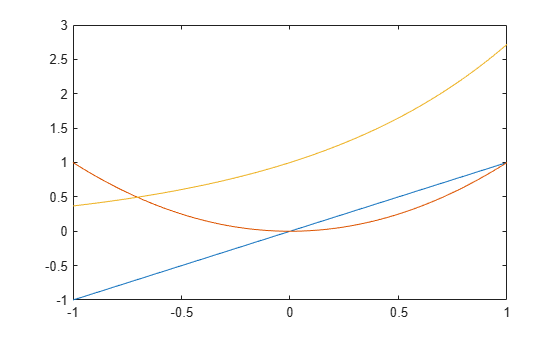Find all objects that have a nonempty `Tag` property.

`h = findobj('-regexp','Tag','[^'']')`
```h = 2x1 Line array: Line (Exponential) Line (Quadratic) ```

Create a vector of four values. Display the values using a line plot, an area plot, and a bar graph.

```y = [1 5 6 3]; subplot(3,1,1) plot(y) subplot(3,1,2) area(y) subplot(3,1,3) bar(y)```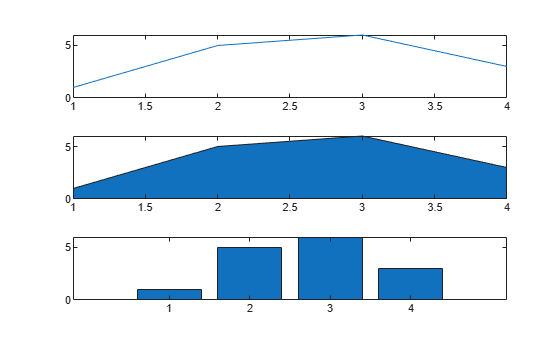Return all objects that have a `BaseValue` property.

`h = findobj('-property','BaseValue')`
```h = 2x1 graphics array: Bar Area ```

Create a plot of random values, and then return all line objects in the current axes.

`plot(rand(5))``h = findobj(gca,'Type','line')`
```h = 5x1 Line array: Line Line Line Line Line ```

Use `h` to query the y values of the first `Line` object.

`values = h(1).YData`
```values = 1×5 0.6557 0.0357 0.8491 0.9340 0.6787 ```

Create a figure with two tabs. Add axes to each tab by specifying the parent container for each one. Plot a line in the first tab and a surface in the second tab.

```figure tab1 = uitab('Title','Tab1'); ax1 = axes(tab1); plot(ax1,1:10) tab2 = uitab('Title','Tab2'); ax2 = axes(tab2); surf(ax2,peaks)```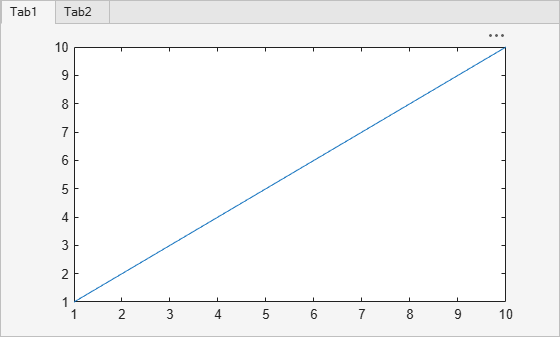Return all objects in the current figure and its descendants.

`h = findobj(gcf)`
```h = 8x1 graphics array: Figure (1) TabGroup Tab (Tab1) Tab (Tab2) Axes Axes Line Surface ```

Create a figure with two stacked subplots.

```subplot(2,1,1) x = linspace(0,10); y1 = sin(x); plot(x,y1) subplot(2,1,2) y2 = sin(5*x); plot(x,y2)```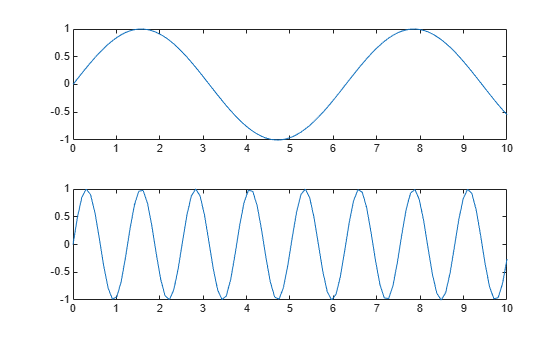Find all objects in the current figure and its children.

`h1 = findobj(gcf,'-depth',1)`
```h1 = 3x1 graphics array: Figure (1) Axes Axes ```

Find all objects in the current figure and any descendants that are up to two levels lower in the graphics object hierarchy.

`h2 = findobj(gcf,'-depth',2)`
```h2 = 5x1 graphics array: Figure (1) Axes Axes Line Line ```

Restrict the search to the current figure and the current axes using the `'flat'` option.

`h3 = findobj([gcf,gca],'flat')`
```h3 = 2x1 graphics array: Figure (1) Axes ```

## Input Arguments

collapse all

Property name, specified as a character vector or string scalar. For more information, see Graphics Object Properties.

Example: `'Tag'`

Example: `'Type'`

Property value, specified as a scalar or array.

Logical operator, specified as `'-and'`, `'-or'`, or `'-xor'`. Logical operator precedence follows MATLAB® precedence rules. For more information, see Operator Precedence.

To control operator precedence, group `prop`,`value` pairs within cell arrays. For example, find all objects that have a `Tag` property set to `'button one'` and a `Color` property set to a value other than `'red'` or `'blue'`:

```h = findobj('Tag','button one','-and', ... '-not',{'Color','red','-or','Color','blue'})```

Regular expression, specified as a string array, character vector, or cell array of character vectors. `expr` can contain characters, metacharacters, operators, tokens, and flags that specify patterns to match in the property value. You can use `expr` only when the property value is a string or character vector. For more information about regular expressions, see `regexp`.

Objects to search from, specified as an array of graphics objects. Unless you specify the `'-depth'` or `'flat'` options, `findobj` searches the objects in the input array `objhandles` and all of their descendants in the graphics object hierarchy.

Depth of search, specified as a nonnegative integer indicating the number of levels below any given object in the input array `objhandles`.

• `d = n` — Search `n` levels of the hierarchy below each object in `objhandles`.

• `d = 0` — Search only the same level as the objects in `objhandles`. This is equivalent to specifying the `'flat'` option.

• `d = inf` — Search all levels below the objects in `objhandles`. This is equivalent to a default search without specifying the `'-depth'` or `'flat'` options.

## Tips

• If the `HandleVisibility` property of an object is set to `'off'`, `findobj` does not return that graphics object or any of its descendants. To return all objects in the hierarchy, including hidden objects, use the `findall` function.

• `findobj` correctly matches any legal property value. For example, this code finds all objects having a `Color` property set to `red`, `r`, or ```[1 0 0]```:

`findobj('Color','r')`

• When a graphics object is a descendant of more than one object identified in `objhandles`, MATLAB searches the object each time `findobj` encounters its handle. Therefore, implicit references to a graphics object can result in multiple returns of the object.

## Version History

Introduced before R2006a